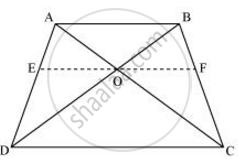# ABCD is a trapezium in which AB || DC and its diagonals intersect each other at the point O. Show that (AO)/(BO) = (CO)/(DO) - Mathematics

ABCD is a trapezium in which AB || DC and its diagonals intersect each other at the point O. Show that (AO)/(BO) = (CO)/(DO)

#### SolutionDraw a line EF through point O, such that EF || CD

By using basic proportionality theorem, we obtain

(AE)/(ED) = (AO)/(OC) ........(1)

In ΔABD, OE || AB

So, by using basic proportionality theorem, we obtain

(ED)/(AE) = (OD)/(BO)

=> (AE)/(ED) = (BO)/(OD)  .....(2)

From equations (1) and (2), we obtain

(AO)/(OC) = (BO)/(OD)

⇒ (AO)/(BO) = (OC)/(OD)

Concept: Similarity of Triangles
Is there an error in this question or solution?

#### APPEARS IN

NCERT Class 10 Maths
Chapter 6 Triangles
Exercise 6.2 | Q 9 | Page 129